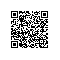# 编程之美——小飞的电梯调度算法之新解（中位数）

1. int controlElevator(int nPerson[], int nfloor, int upWeight){
2.     int targetFloor = 1;
3.     int minFloor = 0;
4.     int N1 = 0;
5.     int N2 = nPerson;
6.     int N3 = 0;
7.     int i = 0;
8.     for(i = 2; i <= nfloor; i++){ //i表示大众意义上的第i层
9.         N3 += nPerson[i];
10.         minFloor += (nPerson[i] * (i - 1) * upWeight);
11.     }
12.     for(i = 2; i <= nfloor; i++){
13.         if(N1 + N2 < N3 * upWeight){
14.             minFloor += (N1 + N2 - N3 * upWeight);
15.             N3 -= nPerson[i];
16.             N1 += N2;
17.             N2 = nPerson[i];
18.             targetFloor = i;
19.         }
20.         else
21.             break;
22.     }
23.     return minFloor;
24. //  return targetFloor;
25. }使用钉钉扫一扫加入圈子
+ 订阅